## Autocatalysis in Reaction Networks

#### Manoj Gopalkrishnan

Since this is my first time writing a blog post here, let me start with a word of introduction. I am a computer scientist at the Tata Institute of Fundamental Research, broadly interested in connections between Biology and Computer Science, with a particular interest in reaction networks. I first started thinking about them during my Ph.D. at the Laboratory for Molecular Science. My fascination with them has been predominantly mathematical. As a graduate student, I encountered an area with rich connections between combinatorics and dynamics, and surprisingly easy-to-state and compelling unsolved conjectures, and got hooked.

There is a story about Richard Feynman that he used to take bets with mathematicians. If any mathematician could make Feynman understand a mathematical statement, then Feynman would guess whether or not the statement was true. Of course, Feynman was in a habit of winning these bets, which allowed him to make the boast that mathematics, especially in its obsession for proof, was essentially irrelevant, since a relative novice like himself could after a moment's thought guess at the truth of these mathematical statements. I have always felt Feynman's claim to be unjust, but have often wondered what mathematical statement I would put to him so that his chances of winning were no better than random.

Today I want to tell you of a result about reaction networks that I have recently discovered with Abhishek Deshpande. The statement seems like a fine candidate to throw at Feynman because until we proved it, I would not have bet either way about its truth. Even after we obtained a short and elementary proof, I do not completely 'see' why it must be true. I am hoping some of you will be able to demystify it for me. So, I'm just going to introduce enough terms to be able to make the statement of our result, and let you think about how to prove it.

John and his colleagues have been talking about reaction networks as Petri nets in the network theory series on this blog. As discussed in part 2 of that series, a Petri net is a diagram like this: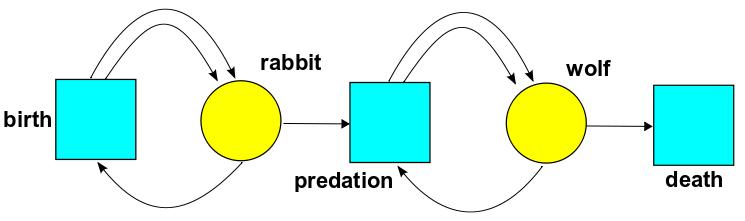Following John's terminology, I will call the aqua squares 'transitions' and the yellow circles 'species'. If we have some number #rabbit of rabbits and some number #wolf of wolves, we draw #rabbit many black dots called 'tokens' inside the yellow circle for rabbit, and #wolf tokens inside the yellow circle for wolf, like this: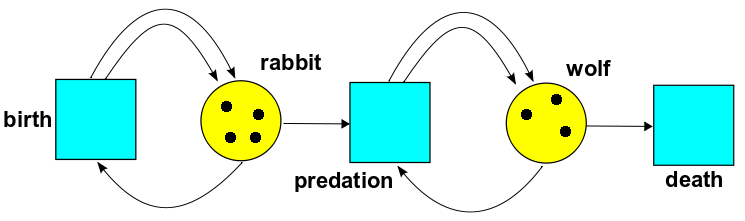Here #rabbit = 4 and #wolf = 3. The predation transition consumes one 'rabbit' token and one 'wolf' token, and produces two 'wolf' tokens, taking us here: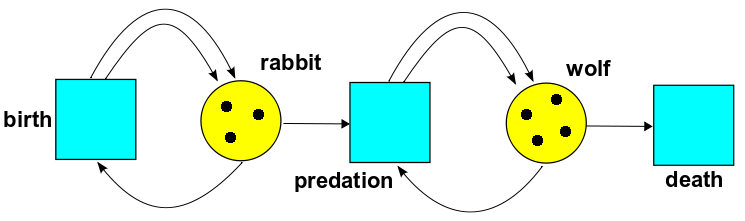John explained in parts 2 and 3 how one can put rates on different transitions. For today I am only going to be concerned with 'reachability:' what token states are reachable from what other token states. John talked about this idea in part 25.

By a complex I will mean a population vector: a snapshot of the number of tokens in each species. In the example above, (#rabbit, #wolf) is a complex. If $y, y'$ are two complexes, then we write

$$y \to y'$$

if we can get from $y$ to $y'$ by a single transition in our Petri net. For example, we just saw that

$$(4,3)\to (3,4)$$

via the predation transition.

Reachability, denoted $\to^*$, is the transitive closure of the relation $\to$. So $y\to^* y'$ (read $y'$ is reachable from $y$) iff there are complexes

$$y=y_0,y_1,y_2,\dots,y_k =y'$$

such that

$$y_0\to y_1\to\cdots\to y_{k-1}\to y_k$$

For example, here $(5,1) \to^* (1, 5)$ by repeated predation.

I am very interested in switches. After all, a computer is essentially a box of switches! You can build computers by connecting switches together. In fact, that's how early computers like the Z3 were built. The CMOS gates at the heart of modern computers are essentially switches. By analogy, the study of switches in reaction networks may help us understand biochemical circuits.

A siphon is a set of species that is 'switch-offable'. That is, if there are no tokens in the siphon states, then they will remain absent in future. Equivalently, the only reactions that can produce tokens in the siphon states are those that require tokens from the siphon states before they can fire. Note that no matter how many rabbits there are, if there are no wolves, there will continue to be no wolves. So {wolf} is a siphon. Similarly, {rabbit} is a siphon, as is the union {rabbit, wolf}. However, when Hydrogen and Oxygen form Water, {Water} is not a siphon.

For another example, consider this Petri net: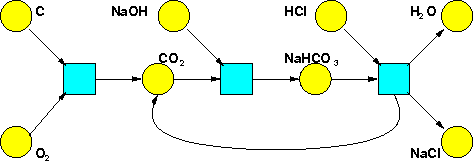The set {HCl, NaCl} is a siphon. However, there is a conservation law: whenever an HCl token is destroyed, an NaCl token is created, so that #HCl + #NaCl is invariant. If both HCl and NaCl were present to begin with, the complexes where both are absent are not reachable. In this sense, this siphon is not 'really' switch-offable. As a first pass at capturing this idea, we will introduce the notion of 'critical set'.

A conservation law is a linear expression involving numbers of tokens that is invariant under every transition in the Petri net. A conservation law is positive if all the coefficients are non-negative. A critical set of states is a set that does not contain the support of a positive conservation law.

For example, the support of the positive conservation law #HCl + #NaCl is {HCl, NaCl}, and hence no set containing this set is critical. Thus {HCl, NaCl} is a siphon, but not critical. On the other hand, the set {NaCl} is critical but not a siphon. {HCl} is a critical siphon. And in our other example, {Wolf, Rabbit} is a critical siphon.

Of particular interest to us will be minimal critical siphons, the minimal sets among critical siphons. Consider this example: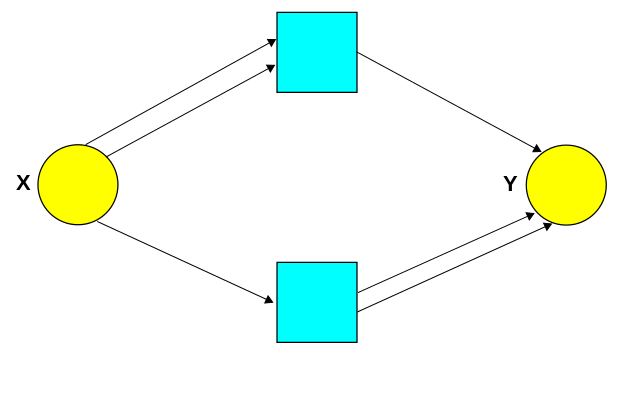Here we have two transitions:

$$X \to 2Y$$

and

$$2X \to Y$$

The set $\{X,Y\}$ is a critical siphon. But so is the smaller set $\{X\}$. So, $\{X,Y\}$ is not minimal.

We define a self-replicable set to be a set $A$ of species such that there exist complexes $y$ and $y'$ with $y\to^* y'$ such that for all $i \in A$ we have

$$y'_i > y_i$$

So, there are transitions that accomplish the job of creating more tokens for all the species in $A$. In other words: these species can 'replicate themselves'.

We define a drainable set by changing the $>$ to a $<$. So, there are transitions that accomplish the job of reducing the number of tokens for all the species in $A$. These species can 'drain away'.

Now here comes the statement:

Every minimal critical siphon is either drainable or self-replicable!

We prove it in this paper:

• Abhishek Deshpande and Manoj Gopalkrishnan, Autocatalysis in reaction networks.

But first note that the statement becomes false if the critical siphon is not minimal. Look at this example again:The set $\{X,Y\}$ is a critical siphon. However $\{X,Y\}$ is neither self-replicable (since every reaction destroys $X$) nor drainable (since every reaction produces $Y$). But we've already seen that $\{X,Y\}$ is not minimal. It has a critical subsiphon, namely $\{X\}$. This one is minimal—and it obeys our theorem, because it is drainable. Checking these statements is a good way to make sure you understand the concepts! I know I've introduced a lot of terminology here, and it takes a while to absorb.

Anyway: our proof that every minimal critical siphon is either drainable or self-replicable makes use of a fun result about matrices. Consider a real square matrix with a sign pattern like this:

$$\left( \begin{array}{cccc} <0 & >0 & \cdots & > 0 \\ >0 & <0 & \cdots &> 0 \\ \vdots & \vdots & <0 &> 0 \\ >0 & >0 & \cdots & <0 \end{array} \right)$$

If the matrix is full-rank then there is a positive linear combination of the rows of the matrix so that all the entries are nonzero and have the same sign. In fact, we prove something stronger in Theorem 5.9 of our paper. At first, we thought this statement about matrices should be equivalent to one of the many well-known alternative statements of Farkas' lemma, like Gordan's theorem.

However, we could not find a way to make this work, so we ended up proving it by a different technique. Later, my colleague Jaikumar Radhakrishnan came up with a clever proof that uses Farkas' lemma twice. However, so far we have not obtained the stronger result in Theorem 5.9 with this proof technique.

My interest in the result that every minimal critical siphon is either drainable or self-replicable is not purely aesthetic (though aesthetics is a big part of it). There is a research community of folks who are thinking of reaction networks as a programming language, and synthesizing molecular systems that exhibit sophisticated dynamical behavior as per specification:

Networks that exhibit some kind of catalytic behavior are a recurring theme among such systems, and even more so in biochemical circuits.

Here is an example of catalytic behavior:

$$A + C \to B + C$$

The 'catalyst' $C$ helps transform $A$ to $B$. In the absence of $C$, the reaction is turned off. Hence, catalysts are switches in chemical circuits! From this point of view, it is hardly surprising that they are required for the synthesis of complex behaviors.

In information processing, one needs amplification to make sure that a signal can propagate through a circuit without being overwhelmed by errors. Here is a chemical counterpart to such amplification:

$$A + C \to 2C$$

Here the catalyst $C$ catalyzes its own production: it is an 'autocatalyst', or a self-replicating species. By analogy, autocatalysis is key for scaling synthetic molecular systems.

Our work deals with these notions on a network level. We generalize the notion of catalysis in two ways. First, we allow a catalyst to be a set of species instead of a single species; second, its absence can turn off a reaction pathway instead of a single reaction. We propose the notion of self-replicable siphons as a generalization of the notion of autocatalysis. In particular, 'weakly reversible' networks have critical siphons precisely when they exhibit autocatalytic behavior. I was led to this work when I noticed the manifestation of this last statement in many examples.

Another hope I have is that perhaps one can study the dynamics of each minimal critical siphon of a reaction network separately, and then somehow be able to answer interesting questions about the dynamics of the entire network, by stitching together what we know for each minimal critical siphon. On the synthesis side, perhaps this could lead to a programming language to synthesize a reaction network that will achieve a specified dynamics. If any of this works out, it would be really cool! I think of how abelian group theory (and more broadly, the theory of abelian categories, which includes categories of vector bundles) benefits from a fundamental theorem that lets you break a finite abelian group into parts that are easy to study—or how number theory benefits from a special case, the fundamental theorem of arithmetic. John has also pointed out that reaction networks are really presentations of symmetric monoidal categories, so perhaps this could point the way to a Fundamental Theorem for Symmetric Monoidal Categories.

And then there is the Global Attractor Conjecture, a long-standing open problem concerning the long-term behavior of solutions to the rate equations. Now that is a whole story by itself, and will have to wait for another day.